Equilibrium (2016 VCE) 1) How does diluting a 0.1 M solution of lactic acid, HC3H5O3, change its pH and percentage ionisation?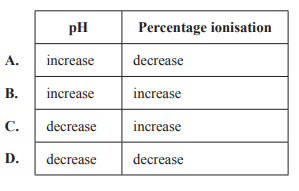Solution2) A chemist injected 0.10 mol carbon monoxide gas, CO, and 0.20 mol chlorine gas, Cl2, into a previously evacuated and sealed 1.0 L flask. At that instant, the following reaction began to occur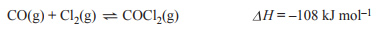The concentrations of the three species present in the flask were monitored over time. The flask was held at a constant temperature. The following concentration–time graph was obtained.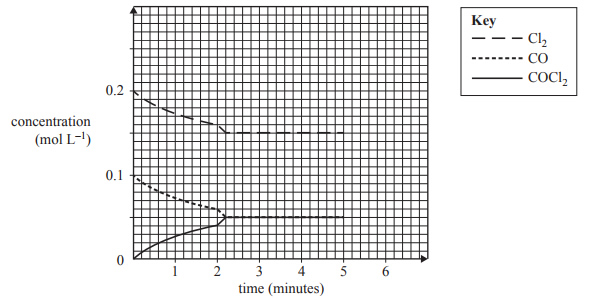a) The most likely sudden change made to the system at the two-minute mark would be that A. a catalyst was injected into the flask. B. the volume of the flask was increased. C. an inert gas was injected into the flask. D. some of the gas mixture was removed from the flask. Solution b) The magnitude of the equilibrium constant for the reaction at the temperature of the experiment is A. 0.15 B. 1.4 C. 3.0 D. 6.7 Solution c) If the equilibrium system were suddenly heated at constant volume at the five-minute mark, which one of the following changes would result? A. The concentration of COCl2 would increase. B. The total gas pressure in the flask would decrease. C. The equilibrium constant for the reaction would increase. D. The total number of gas molecules in the flask would increase Solution3) A class of Chemistry students investigated the reaction of copper metal and iodine solution. After making predictions about the reaction, they placed a copper strip into an iodine solution and compared their predictions with their observations. A number of groups recorded the following.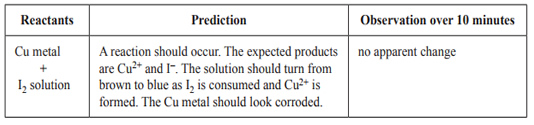The predicted results were not observed. The class was asked to suggest some hypotheses to explain the unexpected result. Which one of the following hypotheses could not explain the unexpected result? A. The reaction rate might have been too slow for the time allowed. B. An equilibrium was established and [Cu2+] was too low to be visible. C. A bromine solution was accidentally used in place of the iodine solution. D. The surface of the copper metal was greasy. Solution4) A team of chemists was investigating the following equilibrium reaction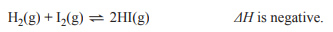Hydrogen gas, H2, and iodine gas, I2, were injected into a sealed container and the mixture was allowed to reach equilibrium. The effect of the following changes on the amount of HI was measured: 1. More H2 gas was injected into the container at a constant temperature and volume. 2. The temperature of the gases was decreased at a constant volume. 3. Some argon gas, Ar, was injected into the container at a constant temperature and volume. 4. The volume of the container was decreased at a constant temperature. Which change(s) would have resulted in the formation of a greater amount, in mol, of HI? A. 1 and 2 only B. 1, 2 and 4 only C. 3 only D. 1 and 4 only Solution5) Bromomethane, CH3Br, is a toxic, odourless and colourless gas. It is used by quarantine authorities to kill insect pests. A simplified reaction for its synthesis is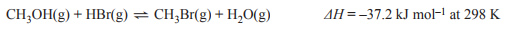The manufacturer of this chemical investigates reaction conditions that could affect the time the process takes and the percentage yield. a. Predict the effect of each change given below on the rate of production of bromomethane and circle your prediction. (increase, no change or decrease). Give your reasoning. • Increasing temperature (constant volume) increase     no change      decrease • Increasing pressure (constant temperature) increase      no change      decrease Solutionb) Considering the system at equilibrium, predict the effect of each change given below on the percentage yield of bromomethane and circle your prediction (increase, no change or decrease). Give your reasoning. • Increasing pressure (constant temperature) increase      no change      decrease • Continuously removing the product CH3Br (constant volume and temperature) increase      no change      decrease Solutionc) The graph below represents the concentration of three of the species involved in the production of CH3Br, when they are at equilibrium at constant temperature. At time t1, a small amount of HBr was suddenly added to the equilibrium mixture. Complete the graph after t1, showing the changes in concentration for each of the three species.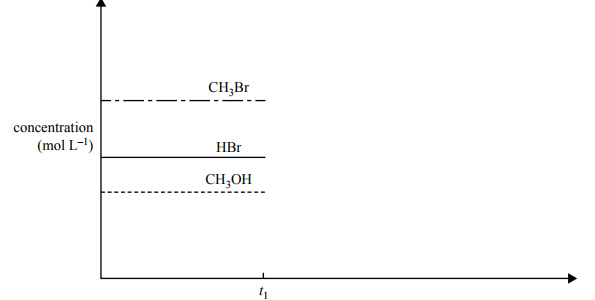Solutiond) When bromomethane is used by quarantine officers, it is pumped into a sealed room that contains the items to be treated. Describe one safety precaution that quarantine officers would need to consider when using bromomethane Solution